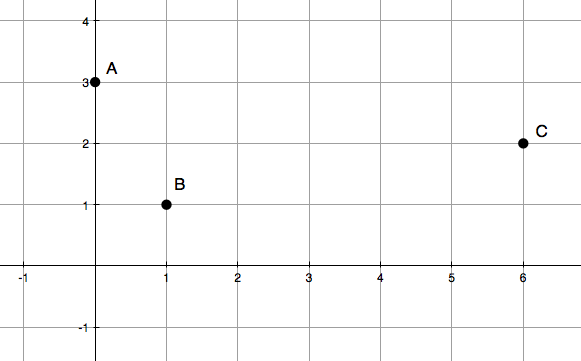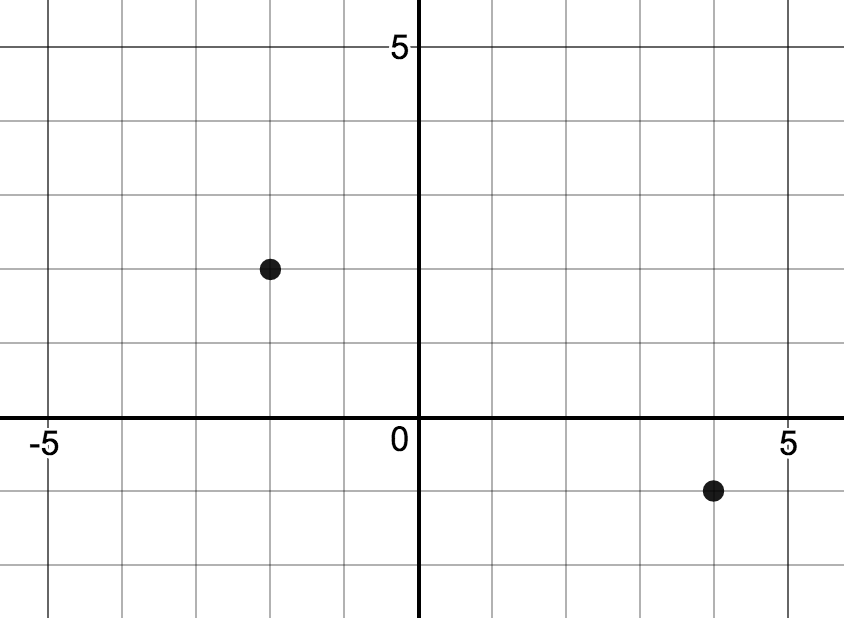Match Fishtank is now Fishtank Learning!

# Linear Equations, Inequalities and Systems

## Objective

Write linear equations given features, points, or graph in standard form, point-slope form, and slope-intercept form.

## Common Core Standards

### Core Standards

?

• F.IF.B.4 — For a function that models a relationship between two quantities, interpret key features of graphs and tables in terms of the quantities, and sketch graphs showing key features given a verbal description of the relationship. Key features include: intercepts; intervals where the function is increasing, decreasing, positive, or negative; relative maximums and minimums; symmetries; end behavior; and periodicity. Modeling is best interpreted not as a collection of isolated topics but in relation to other standards. Making mathematical models is a Standard for Mathematical Practice, and specific modeling standards appear throughout the high school standards indicated by a star symbol (★). The star symbol sometimes appears on the heading for a group of standards; in that case, it should be understood to apply to all standards in that group.

• F.IF.C.7.A — Graph linear and quadratic functions and show intercepts, maxima, and minima.

• A.SSE.B.3 — Choose and produce an equivalent form of an expression to reveal and explain properties of the quantity represented by the expression. Modeling is best interpreted not as a collection of isolated topics but in relation to other standards. Making mathematical models is a Standard for Mathematical Practice, and specific modeling standards appear throughout the high school standards indicated by a star symbol (★). The star symbol sometimes appears on the heading for a group of standards; in that case, it should be understood to apply to all standards in that group.

?

• 8.EE.B.5

• 8.EE.B.6

## Criteria for Success

?

1. Rearrange a linear equation to reveal the slope when an equation is given in standard form.
2. Substitute zeros for the appropriate variable to find the intercepts of a linear function.
3. Write a linear equation given features of the linear equation.
4. Graph a linear equation from all three forms of linear equation.

## Tips for Teachers

?

Ensure that students have the general forms of all three major forms of a linear equation (standard, slope-intercept, point-slope) in their notes by the end of this lesson.

## Anchor Problems

?

### Problem 1

Prove algebraically that point $C$ is not on the same line as points $A$ and $B$#### References

Put the Point on the Line by Desmos is made available by Desmos. Copyright © 2017 Desmos, Inc. Accessed Oct. 19, 2017, 2:32 p.m..

Modified by Fishtank Learning, Inc.

### Problem 2

Graph the linear equation below.

${2x-3y=9}$

### Problem 3

Write a linear equation that contains the points shown in the graph below.## Problem Set

?

The following resources include problems and activities aligned to the objective of the lesson that can be used to create your own problem set.

A linear equation has a slope of $2$ and contains the point ${(-3,5).}$